U.S. Department of Transportation
1200 New Jersey Avenue, SE
Washington, DC 20590
202-366-4000

Federal Highway Administration Research and Technology
Coordinating, Developing, and Delivering Highway Transportation InnovationsThis report is an archived publication and may contain dated technical, contact, and link information
 Federal Highway Administration > Publications > Research Publications > 04097 > Measured Variability Of Southern Yellow Pine - Manual for LS-DYNA Wood Material Model 143
Publication Number: FHWA-HRT-04-097
Date: August 2007

# Measured Variability Of Southern Yellow Pine - Manual for LS-DYNA Wood Material Model 143

PDF files can be viewed with the Acrobat® Reader®

### 2.4 MODEL FORMULATION

Elastic Constitutive Equations

The general constitutive relationship for an orthotropic material, written in terms of the principal material directions, is:(16)

The subscripts 1, 2, and 3 refer to the longitudinal, tangential, and radial stresses and strains (s1 = s11, s2 = s22, s3 = s33, e1 = e11, e2 = e22, and e3 = e33, respectively). The subscripts 4, 5, and 6 are in a shorthand notation that refers to the shearing stresses and strains (s4 = s12, s5 = s23, s6 = s13, e4 = e12, e5 = e23, and e6 = e13). As an alternative notation for wood, it is common to substitute L (longitudinal) for 1, R (radial) for 2, and T (tangential) for 3. The components of the constitutive matrix, Cij, are listed here in terms of the nine independent elastic constants of an orthotropic material:

The following identity, relating the dependent (minor Poisson’s ratios v21, v31, and v32) and independent elastic constants, is obtained from symmetry considerations of the constitutive matrix:

One common assumption is that wood materials are transversely isotropic. This means that the properties in the tangential and radial directions are modeled the same (i.e., E22 = E33, G12 = G13, and v12 = v13). This reduces the number of independent elastic constants to five: E11, E22, v12, G12, and G23. Furthermore, the Poisson’s ratio in the isotropic plane, v23, is not an independent quantity. It is calculated from the isotropic relationship v  = (E2G)/2G, where E = E22 = E33 and G = G23. Transverse isotropy is a reasonable assumption because the difference between the tangential and radial properties of wood (particularly southern yellow pine and Douglas fir) is small in comparison with the difference between the tangential and longitudinal properties.

Yield Surfaces

The yield surfaces parallel and perpendicular to the grain are formulated from six ultimate strength measurements obtained from uniaxial and pure-shear tests on wood specimens:

 XT Tensile strength parallel to the grain XC Compressive strength parallel to the grain YT Tensile strength perpendicular to the grain YC Compressive strength perpendicular to the grain S|| Shear strength parallel to the grain S┴ Shear strength perpendicular to the grain

The formulation is based on the work of Hashin.(27)

Parallel Modes

For the parallel modes, the yield criterion is composed of two terms involving two of the five stress invariants of a transversely isotropic material. These invariants are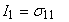and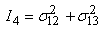. This criterion predicts that the normal and shear stresses are mutually weakening (i.e., the presence of shear stress reduces the strength below that measured in the uniaxial stress tests). Yielding occurs when f|| ≥ 0, where:

Perpendicular Modes

For the perpendicular modes, the yield criterion is also composed of two terms involving two of the five stress invariants of a transversely isotropic material. These invariants are I2 = s22 + s33 and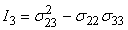. Yielding occurs when f^ ≥ 0, where:

Each yield criterion is plotted in three dimensions in figure 26 in terms of the parallel and perpendicular stresses. Each criterion is a smooth surface (no corners).

Plastic Flow

The plasticity algorithms limit the stress components once the yield criteria in equations 111 and 112 are satisfied. This is done by returning the trial elastic stress state back to the yield surface. The stress and strain tensors are partitioned into elastic and plastic parts. Partitioning is done with a return mapping algorithm that enforces the plastic consistency condition. Separate plasticity algorithms are formulated for the parallel and perpendicular modes by enforcing separate consistency conditions. No input parameters are required.

Figure 26.

Yield criteria for wood produce smooth surfaces in stress space.

Hardening

Wood exhibits prepeak nonlinearity in compression parallel and perpendicular to the grain. Separate translating yield surface formulations are modeled for the parallel and perpendicular modes, which simulate gradual changes in moduli. Each initial yield surface hardens until it coincides with the ultimate yield surface, as shown in figure 27. The initial location of the yield surface determines the onset of plasticity. The rate of translation determines the extent of nonlinearity.

Figure 27.

Prepeak nonlinearity in compression is modeled with translating yield surfaces that allow user to specify hardening response.

For each mode (parallel and perpendicular), the user inputs two parameters―the initial yield surface location in uniaxial compression, N, and the rate of translation, c. If the user wants prepeak nonlinearity to initiate at 70 percent of the peak strength, then the user would input N = 0.3, so that 1 – N = 0.7. If the user wants rapid hardening, then a large value of c is input (e.g., c = 1000). If the user wants gradual hardening, then a small value of c is input (e.g., c = 10).

The state variable that defines the translation of the yield surface is known as backstress and is denoted by aij. Hardening is modeled in compression, but not shear, so the only backstress required for the parallel modes is a11. The value of the backstress is a11 = 0 upon initial yield and a11 = –N|| Xc at ultimate yield (in uniaxial compression). The maximum backstress occurs at ultimate yield and is equal to the total translation of the yield surface in stress space. The backstress components required for the perpendicular modes are a22 and a33. The value of the backstress sum is a22 + a33 = 0 upon initial yield and a22 + a33 = –N^ Yc at ultimate yield (biaxial compression without shear).

Damage

Separate damage formulations are modeled for the parallel and perpendicular modes. These formulations are loosely based on the work of Simo and Ju.(21) If failure occurs in the parallel modes, then all six stress components are degraded uniformly. This is because parallel failure is catastrophic and will render the wood useless. If failure occurs in the perpendicular modes, then only three perpendicular stress components are degraded. This is because perpendicular failure is not catastrophic; the wood may continue to carry load in the parallel direction. Based on these assumptions, the following degradation model is implemented:

Here, each scalar damage parameter, d, transforms the stress tensor associated with the undamaged state,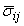, into the stress tensor associated with the damaged state, sij. The stress tensoris calculated by the plasticity algorithm (including viscoplasticity) prior to application of the damage model. Each damage parameter ranges from zero for no damage to approaching unity for maximum damage. Thus, 1 – d is a reduction factor associated with the amount of damage. Each damage parameter evolves as a function of a strain energy-type term. Mesh-size dependency is regulated via a length scale based on the element size (cube root of volume). Damage-based softening is brittle in tension, less brittle in shear, and ductile (no softening) in compression, as demonstrated in figure 28.

Element erosion occurs when an element fails in the parallel mode and the parallel damage parameter exceeds d|| = 0.99. Elements do not automatically erode when an element fails in the perpendicular mode. A flag is available that, when set, allows elements to erode when the perpendicular damage parameter exceeds d^ = 0.99. Setting this flag (IFAIL) is not recommended unless excessive perpendicular damage is causing computational difficulties.

Figure 28.

Softening response modeled for parallel modes of southern yellow pine.

Rate Effects

Data available in the literature for pine indicate that dynamic strength enhancement is more pronounced in the perpendicular direction than in the parallel direction.(16) Therefore, separate rate-effect formulations are modeled for the parallel and perpendicular modes. The formulations increase strength with increasing strain rate by expanding each yield surface:

Parallel

Perpendicular

 where: X and Y =  static strengths X DYNAMIC and Y DYNAMIC =  dynamic strengths C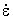(1-n)h =  excess stress components

The excess stress components depend on the value of the fluidity parameter, h; the stiffness, C; and the effective strain rate,. When rate effects are requested via the flag IRATE = 1, the dynamic strengths are used in place of the static strengths in the yield surface formulations.

Setting n > 0 allows the user to model a nonlinear variation in dynamic strength with strain rate. Setting n = 0 allows the user to model a linear variation in dynamic strength with strain rate.

Federal Highway Administration | 1200 New Jersey Avenue, SE | Washington, DC 20590 | 202-366-4000
Turner-Fairbank Highway Research Center | 6300 Georgetown Pike | McLean, VA | 22101